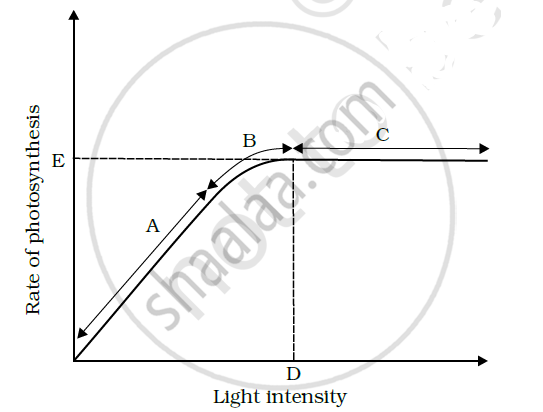CBSE (Science) Class 11CBSE
Share

# In the Figure Shows the Effect of Light on the Rate of Photosynthesis. Based on the Graph, Answer the Following Question - CBSE (Science) Class 11 - Biology

ConceptFactors Affecting Photosynthesis

#### Question

In the Figure shows the effect of light on the rate of photosynthesis. Based on the graph, answer the following questions:

(a) At which point/s (A, B or C) in the curve is light a limiting factor?

(b) What could be the limiting factor/s in region A?

(c) What do C and D represent on the curve?#### Solution(a) Generally, light is not a limiting factor. It becomes a limiting factor for plants growing in shade or under tree canopies. In the given graph, light is a limiting factor at the point where photosynthesis is the minimum. The least value for photosynthesis is in region A. Hence, light is a limiting factor in this region.

(b) Light is a limiting factor in region A. Water, temperature, and the concentration of carbon dioxide could also be limiting factors in this region.

(c) Point D represents the optimum point and gives the light intensity at which the maximum photosynthesis is recorded. The rate of photosynthesis remains constant after this point, even though the intensity of light increases in region C.

Is there an error in this question or solution?

#### APPEARS IN

NCERT Solution for Biology Textbook for Class 11 (2018 to Current)
Chapter 13: Photosynthesis in Higher Plants
Q: 8 | Page no. 225

#### Video TutorialsVIEW ALL 

Solution In the Figure Shows the Effect of Light on the Rate of Photosynthesis. Based on the Graph, Answer the Following Question Concept: Factors Affecting Photosynthesis.
S